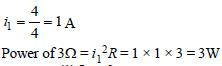Courses

# Test: Current Electricity 2 - From Past 28 Years Questions

## 30 Questions MCQ Test Physics 28 Years Past year papers for NEET/AIPMT Class 12 | Test: Current Electricity 2 - From Past 28 Years Questions

Description
This mock test of Test: Current Electricity 2 - From Past 28 Years Questions for Class 12 helps you for every Class 12 entrance exam. This contains 30 Multiple Choice Questions for Class 12 Test: Current Electricity 2 - From Past 28 Years Questions (mcq) to study with solutions a complete question bank. The solved questions answers in this Test: Current Electricity 2 - From Past 28 Years Questions quiz give you a good mix of easy questions and tough questions. Class 12 students definitely take this Test: Current Electricity 2 - From Past 28 Years Questions exercise for a better result in the exam. You can find other Test: Current Electricity 2 - From Past 28 Years Questions extra questions, long questions & short questions for Class 12 on EduRev as well by searching above.
QUESTION: 1

### In a metre-bridge, the balancing length from theleft end when standard resistance of 1 Ω is inright gap is found to be 20 cm. The value ofunknown resistance is 

Solution:

Let unknown resistance be X. Then
condition of Wheatstone's bridge gives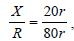where r is resistance of wire
per cm.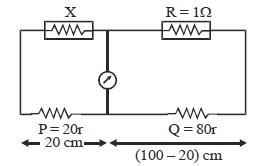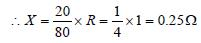QUESTION: 2

### Two electric bulbs, one of 200 V, 40W and otherof 200 V, 100W are connected in a domesticcircuit. Then 

Solution: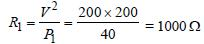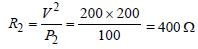∴ R1 (for 40W) > R2 (for 100 W)

QUESTION: 3

### In electrolysis, the amount of mass deposited orliberated at an electrode is directly proportionalto 

Solution:

Amount deposited (m) = Zit = Zq
m ∝ q
Amount deposited is directly proportional
to charge.

QUESTION: 4

Potentiometer measures potential moreaccurately because 

Solution:

Potentiometer measures potential current
more accurately because it measure potential
in open circuit and hence error in potential
due to internal resistance is removed.

QUESTION: 5

Five resistances have been connected as shown in the figure. The effective resistance between A and B is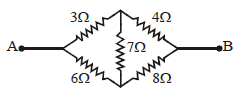Solution:

It is a balanced wheatstone bridge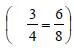, so the 7Ω resistance is
ineffective.
Equivalent resistance of 3Ω and 4Ω = 3 + 4
= 7Ω (series)
Equivalent resistance of 6Ω and 8Ω = 6 + 8
=14Ω (series)
Equivalent resistance of 7Ω and 14Ω (parallel)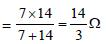QUESTION: 6

A car battery has e.m.f. 12 volt and internalresistance 5 × 10–2 ohm. If it draws 60 amp current,the terminal voltage of the battery will be   

Solution:

E = V + Ir
12 = V + 60 × 5 × 10–2
12 = V + 3
⇒ V = 9 volt

QUESTION: 7

Si and Cu are cooled to a temperature of 300 K,then resistivity? 

Solution:

For metals specific resistance decrease with decrease in temperature where as for semiconductors specific resistance increases with decrease in temperature.

QUESTION: 8

If 25W, 220 V and 100 W, 220 V bulbs areconnected in series across a 440 V line, then    

Solution:

As for an electric appliance R = (Vs2 /W) ,
so for same specified voltage Vs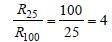i.e, R25 = 4R with R100 = R
Now in series potential divides in proportion
to resistance.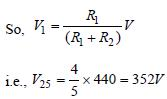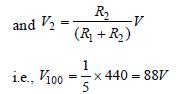From this, it is clear that voltage across 100
W bulb (= 88 V) is lesser than specified
(220 V) while across 25 W bulb (= 352 V) is
greater than specified (220 V), so, 25 W bulb
will fuse.

QUESTION: 9

A battery of 10 V and internal resistance 0.5Ω isconnected across a variable resistance R. Thevalue of R for which the power delivered ismaximum is equal to [1992, 2001]

Solution:

Power is maximum when r = R, R = r = 0.5Ω.

QUESTION: 10

A wire has a resistance of 3.1Ω at 30ºC and aresistance 4.5Ω at 100ºC. The temperaturecoefficient of resistance of the wire 

Solution:

R1 = 3.1 Ω at t = 30°C
R2 = 4.5 Ω at t = 100°C
We have, R = R0 (1 + αt)
∴ R1 = R0 [1 + α (30)]
R2 = R0 [1 + α (100)]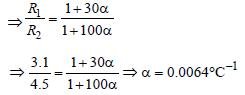QUESTION: 11

If specific resistance of a potentiometer wire is10–7 Ωm, the current flow through it is 0.1 A andthe cross-sectional area of wire is 10–6 m2 thenpotential gradient will be 

Solution:

Potential gradient = Potential fall per unit
length. In this case resistance of unit length.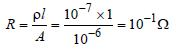Potential fall across R is

V = I.R = 0.1x10-1 = 0.01 volt/m.
= 10-2 volt /m

QUESTION: 12

The resistivity (specific resistance) of a copperwire 

Solution:

Resistivity of copper wire increases with
increase in temprature as ρt = ρ0 (1+ αt)
Copper being a metal has positive coefficient
of resistivity.

QUESTION: 13

The potential difference between the terminals of a cell in an open circuit is 2.2 V. When a resistor of 5Ω is connected across the terminals of the cell, the potential difference between the terminals of the cell is found to be 1.8 V. The internal resistance of the cell is 

Solution:

E = V + ir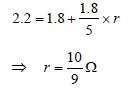QUESTION: 14

An electric kettle has two heating coils. Whenone of the coils is connected to an a.c. source,the water in the kettle boils in 10 minutes. Whenthe other coil is used, the water boils in 40minutes. If both the coils are connected inparallel, the time taken by the same quantity ofwater to boil will be    

Solution: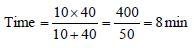QUESTION: 15

Two 220 volt, 100 watt bulbs are connected firstin series and then in parallel. Each time thecombination is connected to a 220 volt a.c.supply line. The power drawn by thecombination in each case respectively will be   

Solution: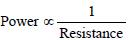In series combination, resistance doubles.

Hence, power will be halved.
In parallel combination, resistance halves.
Hence, power will be doubled.

QUESTION: 16

Fuse wire is a wire of    

Solution:

Fuse wire : It is used in a circuit to control the
maximum current flowing in circuit. It is a thin
wire having high resistance and is made up
of a material with low melting point.

QUESTION: 17

In a Wheatstone’s bridge all the four arms have equal resistance R. If the resistance of the galvanometer arm is also R, the equivalent resistance of the combination as seen by the battery is    

Solution:

Since, Wheatstone's bridge is balanced, then
resistance of galvanometer will be
uneffective.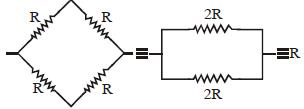QUESTION: 18

A battery is charged at a potential of 15V for 8hours when the current flowing is 10A. Thebattery on discharge supplies a current of 5Afor 15 hours. The mean terminal voltage duringdischarge is 14V. The “watt-hour” efficiency ofthe battery is 

Solution:

Efficiency is given by η = output/input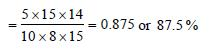QUESTION: 19

When three identical bulbs of 60 watt, 200 voltrating are connected in series to a 200 voltsupply, the power drawn by them will be        

Solution: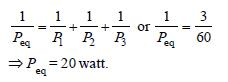QUESTION: 20

In India electricity is supplied for domestic useat 220 V. It is supplied at 110 V in USA. If theresistance of a 60 W bulb for use in India is R,the resistance of a 60 W bulb for use in USA will  be    

Solution: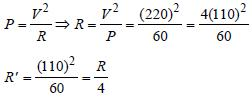QUESTION: 21

The electric resistance of a certain wire of iron isR. If its length and radius are both doubled, then

Solution: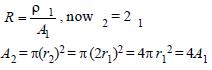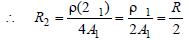∴ Resistance is halved, but specific
resistance remains the same.

QUESTION: 22

Resistances n, each of r ohm, when connectedin parallel give an equivalent resistance of Rohm. If these resistances were connected inseries, the combination would have a resistancein ohms, equal to 

Solution: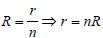When connected in series, Req = nr
= n (nR) = n2R

QUESTION: 23

Five equal resistances each of resistance R are connected as shown in the figure. A battery of V volts is connected between A and B. The current flowing in AFCEB will be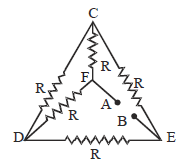Solution:

A balanced Wheststone’s bridge exists
between A & B.

∴ Req = R
Current through circuit = V/R
Current through AFCEB = V/2R

QUESTION: 24

A 6 volt battery is connected to the terminals ofthe three metre long wire of uniform thicknessand resistance of 100 ohm. The difference ofpotential between two points on the wireseparated by a distance of 50 cm will be  

Solution:

R α
For 300 cm, R = 100 Ω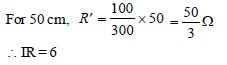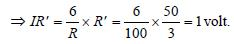QUESTION: 25

A 5–ampere fuse wire can withstand amaximum power of 1 watt in the circuit. Theresistance of the fuse wire is 

Solution: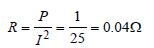QUESTION: 26

When a wire of uniform cross–section a, length l and resistance R is bent into a complete circle, resistance between any two
of diametrically opposite points will be 

Solution: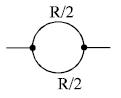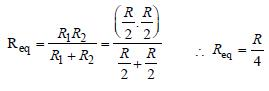QUESTION: 27

For the network shown in the Fig. the value of the current i is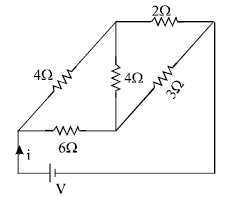Solution:

It is a balanced Wheatstone bridge. Hence
resistance 4Ω can be eliminated.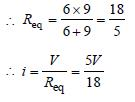QUESTION: 28

Two batteries, one of emf 18 volt and internal resistance 2Ω and the other of emf 12 volt andinternal resistance 1Ω, are connected asshown. The voltmeter V will record a readingof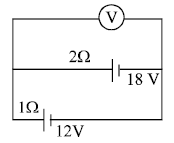Solution: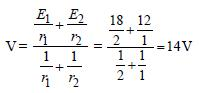(Since the cells are in parallel).

QUESTION: 29

In producing chlorine through electrolysis, 100watt power at 125 V is being consumed. Howmuch chlorine per minute is liberated? E.C.E. ofchlorine is 0.367 × 10–6 kg/ coulomb. 

Solution:

Power = V × I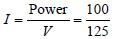E.C.E. of chlorine is 0.367 × 10–6 kg/coulomb
Charge passing in one minute =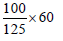= 48 coulomb

Chlorine precipitated = 0.367 × 10–6 × 48
= 17.6 × 10–6 kg
= 17.6 mg

QUESTION: 30

Power dissipated across the 8Ω resistor in the circuit shown here is 2 watt. The power dissipated in watt units across the 3Ω resistor is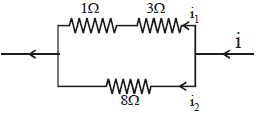Solution:

Power = V . I = I2R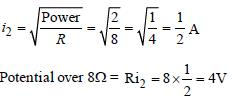This is the potential over parallel branch. So,

i1 = 4/4 =1A